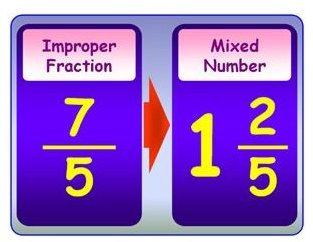# Learn About Improper Fractions and How to Reduce Them

Page content

Knowing what an improper fraction is will help you better understand fractions, and the definition is not too complicated. An improper fraction is a fraction that has a numerator (top number) that is equal to or greater than its denominator (bottom number).

When an improper fraction has the same number on the top and bottom, it is equal to 1. However, if an improper fraction has a number on top that is larger than the bottom number it becomes a mixed fraction when it gets simplified. You may have an improper fraction when you are adding or subtracting fractions, but need to reduce an improper fraction when you are finished to get your final answer. We’ll explain how.

## Reducing FractionsFirst, divide the numerator by the denominator. We will use 11/4 in our example. Take 11 and divide it by 4. 4 does not go into 11 an equal number of times but does twice.

Write down the whole number that we get in step one. Since 4 goes into 11 two times, write down 2. This is the whole number part of the fraction.

Write down how much is left of the numerator after you divide in step one. 4 goes into 11 two times to equal 8. Take 11 and subtract 8 to get 3.

Write down the remainder left over in your improper fraction and place it over your denominator. In our example, we have 3/4. Our final answer is 11/4 is equal to 2 3/4. (The image to the right shows 7/5 reduced to 1 2/5.)

## Adding Mixed Numbers and FractionsAlthough reducing an improper fraction is a useful skill, you are going to see improper fractions more often when you need to add and subtract fractions with mixed numbers. For this example, we are going to add two mixed numbers together and then reduce an improper fraction to get our final answer. This example may help you understand the concept of what an improper fraction is and how it applies to real problems in mathematics.

For our example, we are going to use the problem 3 2/3 + 5 2/3. This is an easier problem to work with because the denominators are the same, which eliminates the need to reduce the fraction to lowest terms. First, add the numerators on both fractions together and place the number over the current denominator. 2/3 + 2/4 = 4/3. We now have an improper fraction.

Convert the improper fraction to a mixed number by dividing 3 by 4. 3 goes into 4 one time. Write down the 1, which is the number part of the new mixed fraction.

Take 4, which is the numerator and subtract 3, since 3 went into 4 once. We have 1 left. Place the 1 over the denominator, which is 3 to get 1/3. Our total is 1 1/3.

Complete the problem by adding the three whole numbers together; the 3 from 3 2/3, 5 from 5 2/3, and the 1 from 4/3 to get a final answer of 9 1/3.

## Practice

Practice makes perfect. You need to practice with multiple problems to understand the concept of what is an improper fraction. Try examples at the following sites to perfect your skills: